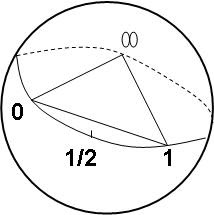Last November, in the pre maths blogger days, we started with Mulase's lectures on moduli spaces of Riemann surfaces. In particular, let us look once more at the $S_3$ action on the Riemann sphere $\mathbb{CP}^1$.The real axis is the equator, with the point $\frac{1}{2}$ sitting opposite the point at infinity. The dihedral action helps define a compactified form of the moduli space for the once punctured torus $M_{1,1}$ (elliptic curve), which was described by a glued region of the upper half plane, sitting above the unit circle. The j invariant gives the mapping from the 3-punctured Riemann sphere to the complex plane which respects the dihedral action on the equatorial triangle, and the torus orbifold is the quotient space.
The j invariant is used to obtain Grothendieck's ribbon diagram from the inverse image of the interval $[0,1]$, so both sphere and torus moduli are essential in understanding the ribbon for the 3-punctured sphere. Recall that these are the only moduli of real dimension 2. In the six dimensions of twistors there are three complex moduli, namely $M_{0,6}$, $M_{1,3}$ and $M_{2,0}$ which have (respectively) orbifold Euler characteristics of $-6$, $- \frac{1}{6}$ and $- \frac{1}{120}$. Octonion analogues of such moduli will be easier to understand using n-operad combinatorics, because non-commutative and non-associative geometry is a tricky business.